The GLM Procedure
 WEIGHT Statement
WEIGHT variable ;

When a WEIGHT statement is used, a weighted residual sum of squares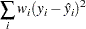is minimized, where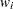is the value of the variable specified in the WEIGHT statement,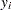is the observed value of the response variable, and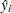is the predicted value of the response variable.

If you specify the WEIGHT statement, it must appear before the first RUN statement or it is ignored.

An observation is used in the analysis only if the value of the WEIGHT statement variable is nonmissing and greater than zero.

The WEIGHT statement has no effect on degrees of freedom or number of observations, but it is used by the MEANS statement when calculating means and performing multiple comparison tests (as described in the section MEANS Statement).

The normal equations used when a WEIGHT statement is present are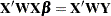where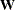is a diagonal matrix consisting of the values of the variable specified in the WEIGHT statement.

If the weights for the observations are proportional to the reciprocals of the error variances, then the weighted least squares estimates are best linear unbiased estimators (BLUE).Previous Page | Next Page | Top of Page